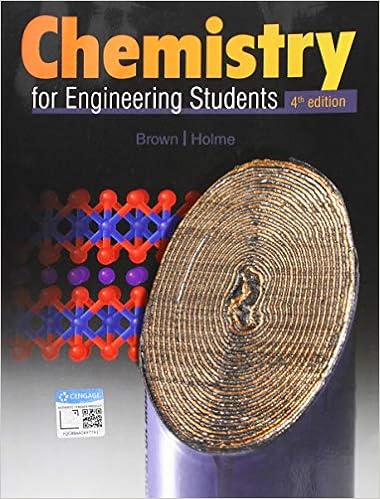# Consider a redox reaction between molecules a and b

• Homework Help
• 5
• 100% (1) 1 out of 1 people found this document helpful

This preview shows page 4 - 5 out of 5 pages.

##### We have textbook solutions for you!
The document you are viewing contains questions related to this textbook.The document you are viewing contains questions related to this textbook.
Chapter 9 / Exercise 9.67
Chemistry for Engineering Students
Brown/HolmeExpert Verified
12.Consider a redox reaction between molecules A and B. Molecule A has a redox potential of 100 mV and molecule B has a redox potential of +100 mV. For the transfer of electrons from A to B, is the G° positive or negative or zero? Under what conditions will the reverse reaction, transfer of electrons from B to A, occur? 13.One of the problems in understanding redox reactions is coming to grips with the language. Consider the reduction of pyruvate by NADH:
##### We have textbook solutions for you!
The document you are viewing contains questions related to this textbook.The document you are viewing contains questions related to this textbook.
Chapter 9 / Exercise 9.67
Chemistry for Engineering Students
Brown/HolmeExpert Verified
HW#9_Fall 2015 NAME: ________________________________ 5
Summing these two half reactions and their Eo` values gives the overall equation and its ∆Eo` value:Pyruvate + NADH + H+lactate + NAD+∆Eo` = +0.13VWhen a redox reaction takes place under nonstandard conditions, the tendency to donate electrons (∆E) is equal to ∆Eo` modified by a concentration term:∆E = ∆Eo` - 2.3𝑅𝑇𝑛𝐹𝑙𝑜𝑔[𝑙𝑎𝑐?𝑎?𝑒][𝑁𝐴𝐷+][𝑝𝑦𝑟??𝑎?𝑒][𝑁𝐴𝐷𝐻]where R = 1.98 x 10-3kcal/Kmole, T=temperature in kelvins, n= the number of electrons transferred, and F = 23 kcal/V mole. ∆G is related to ∆E by the equation:G = -nF∆ESince the signs of ∆G and ∆E are opposite, a favorable redox reaction has a positive ∆E and a negative ∆G.a.Calculate ∆G for reduction of pyruvate to lactate at 37oC, with all reactant and products at a concentration of 1 M. b.Calculate ∆G for the reaction at 37oC under conditions where the concentrations of pyruvate and lactate are equal and the concentrations of NAD+ and NADH are equal. c.What would the concentration term need to be for this reaction to have a ∆G of zero at 37oC? d.Under normal conditions in vascular smooth muscle (at 37oC), the concentration ratio of NAD+ to NADH is 1000, the concentration of lactate is 0.77 μmol/g, and the concentration of pyruvate is 0.15 µmol/g. What is ∆G for reduction of pyruvate to lactate under these conditions?
•••